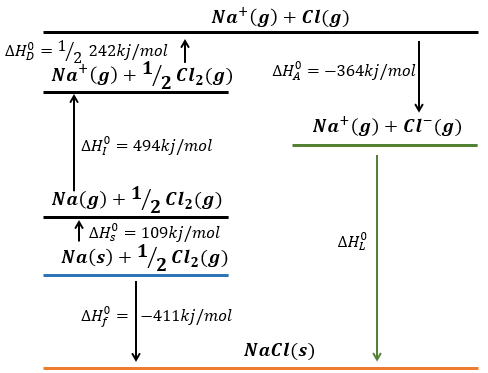# Chapter 4d: Solids – lattice energy

In gases, the heat capacity was a resultant of translation, vibration and rotation.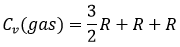In a solid, there is no translation and no rotation. The atoms may vibe around their equilibrium position. The vibration involves a potential energy and a kinetic energy, each term participating for ½R. As a solid has 3 dimensions, Cv=3R

However, this equality is not true at low temperatures. It is related to the radiation of the black body. In this case, the heat capacity is given by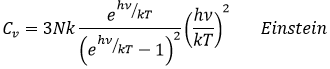A black body is an idealised body that absorbs all electromagnetic radiation (of any frequency). It only emits a radiation called black-body radiation that depends on the temperature alone. A model for the black body is a cavity with a tiny opening.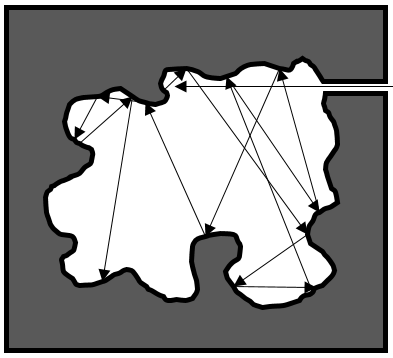The radiation penetrating in the cavity are trapped inside because of tis geometry and/or the absorption of the radiation by the walls of the cavity.

At a fixed temperature and considering the thermal equilibrium, the hole may allow some radiation to escape from the black body. It is the black-body radiation and it only depends on the temperature. Because of the size of the hole with regard to the body, the escaping radiation has negligible effect upon the equilibrium of the radiation inside the cavity.

The reticular energy U0, or energy of network is the energy of the solid at T=0K. The energy of the solid at a given temperature is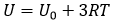We cannot measure U0 but we can approximate its value from the enthalpy of sublimation: to determine the energy of the solid, we break all the liaisons to obtain the gas.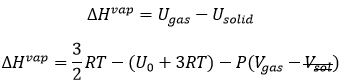The last term comes from the dilatation of the solid into the gas. The volume of the solid is negligible with regard to the volume of the gas. If we consider a perfect gas, then pV=RT. As a result,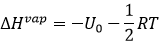We can also find the reticular energy with the Born-Haber cycle explained in a previous section.

For a solid with several elements, such as an ionic solid, the calculation is more complex: there are two or more gases and electronic interactions (ionic liaisons, repulsion).

Born–Haber cycle

Atoms form solids because it leads to a decrease of their energy. One measure to quantify this stabilisation is the lattice energy ΔH0L, i.e. the energy required to form a solid from the gaseous ions.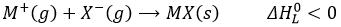This energy is negative and cannot be determined experimentally. The usual way to determine it is to build the so-called Born–Haber cycle. This cycle is made from the basic transformations of the constituents, taken them apart one from each other and starting from their normal state at sctp. Several transformations can be considered:

• Sublimation: transformation from a solid to a gas
• Vaporisation: transformation from a liquid to a gas
• Electroaffinity: the addition of an electron to a gas particle
• Dissociation: the separation of two atoms
• Ionisation: the liberation of an electron from a substrate

Let’s build the Born–Haber cycle for NaCl. We want to determine the enthalpy of this reaction: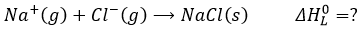To do so, we start from a reaction with a known enthalpy. We know that the enthalpy of reaction to form the solid from Na(s) and Cl2(g) is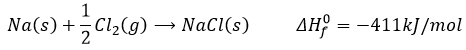The transformation from Na (s) to Na+(g) is made in two steps: first the sublimation of the solid into a gas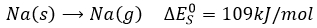And then the ionisation of the gas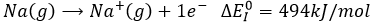There are two steps as well to obtain Cl(g) from Cl2(g). The first step is the dissociation of the two atoms of Cl2. As we only need one atom of chlorine, the enthalpy of dissociation is divided by 2.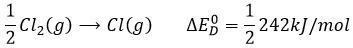The second step is to add an electron to Cl(g) (electroaffinity)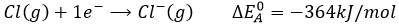This energy is heavily negative because the chlorine reaches the octet. Now we have the two gaseous ions. We just need to add the enthalpy of all the steps together to obtain the lattice energy. The Born–Haber cycle is often represented this way: